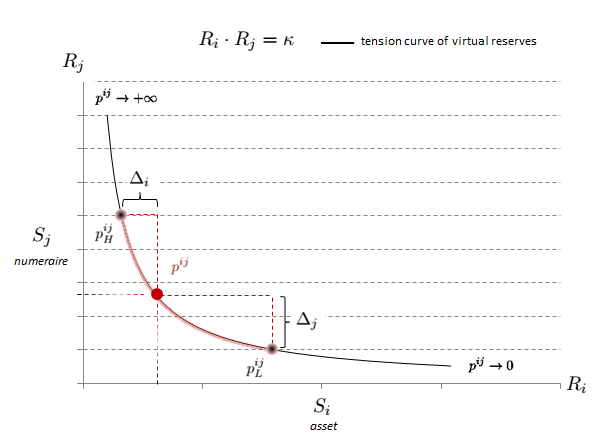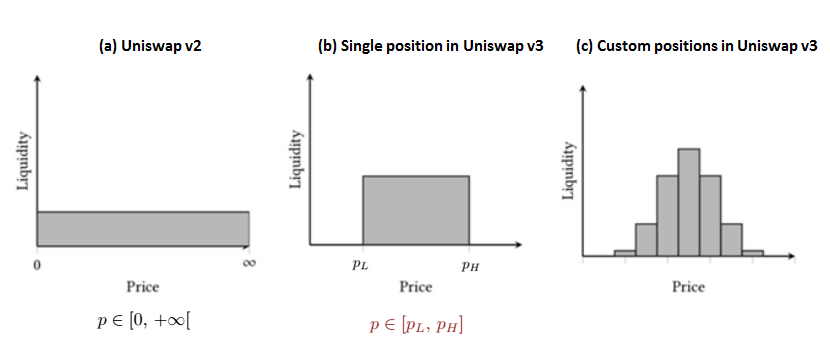Sep 19, 2022

# Mathematical Surgery of AMM - Part 2

This document is the second part of mathematical surgery of automatic market makers DeFi protocols. It summarizes all the LinkedIn posts from $#6a$ to $#6c$.

## Uniswap-v3

### Liquidity providers, an illustration

The earlier versions of the AMM protocol aimed to provide liquidity across the entire possible price range $0 \leq p_{t} < +\infty$ as it is illustrated by the graph below:Figure 1: Liquidity Function for Uniswap v2

Let’s suppose that the actual price of the token pair fluctuates within a range $[p^{ij}{L}, p^{ij}{H}]$, then moving from the current price $p^{ij}$ to a lower price $p^{ij}{L}$ would consume the maximal amount of reserve $\Delta_j$ of token $S_j$, while moving to a higher price $p^{ij}{H}$ would consume the maximal amount of reserve $\Delta_i$ of token $S_i$. Under this fluctuation hypothesis, it is just necessary to provide the two ‘reserves’ $\Delta_i$ and $\Delta_j$, as the capital $Ri-\Delta_i$ and $R_j-\Delta_i$ is not exploited.

If this bonding curve or tension curve is simple to implement and allows liquidity to be efficiently aggregated, there is nonetheless a design issue: much of the assets held in a pool are never employed. Besides, it is important to notice that in Uniswap-v1, it was necessary to send the numeraire-token $S_j$ before receiving the asset-token $S_i$ bought in the swap, and this constraint made many arbitrages rather impossible to achieve[^1]. To resolve this issue, Uniswap-v2 brought a solution by allowing a user to receive and use the token $S_i$ before paying for it and sending the token $S_j$ as long as they make the payment within the same atomic transaction.

Before diving deep into the Uniswap-v3 intricacy, it is useful to build a practical intuition about how the liquidity protocol works. For the sake of illustration, let’s consider the liquidity pool $\mathcal{L}^{ETH,DAI}$: a classical investment strategy run by a liquidity provider (LP) is to provide two reserves $R_{\text{DAI}}$ and $R_{\text{ETH}}$ of tokens - so that the AMM protocol can function as a decentralized autonomous market place - in exchange of a portion of the transaction fees generated by the trading activity in the pool, which is proportional to their personal allocation with respect to the pool’s size. This investment strategy seems to belong to a mere digital money market primitive. Actually, as it will be proved latter, it is more exactly a ‘derivatives’ investment strategy written on this primitive. In exchange for putting up their funds, this LP receives a liquidity token that represents his contribution to the pool: this token is redeemed for a share of the trading fees; whenever this LP decides to exit, he receives a portion of the total fees from the reserve relative to the staked amount in that pool. The liquidity token they received keeps a record of owed reserves that have been staked and is then burnt.

Uniswap-v3 allows them to select a level of transaction fees: 0.001% for very stable pairs, 0.05% for fairly stable pair, 0.30% for most pairs and 1.00% for exotic pairs. Because this liquidity pool $\mathcal{L}$ is rather stable, the chosen transaction fee is likely $r=0.05%$.

The critical improvement comes from the principle of concentrated liquidity that allows every LP to allocate their ‘investment’ over a single or many custom exchange price ranges:Figure 2: Liquidity allocation on Uniswap v2 and v3

If Uniswap-v2 provides the same liquidity on all price points, Uniswap-v3 liquidity is made up from liquidity on a series of different positions, the liquidity near current price would be higher than the others.

So let’s assume that the price of the ETH-token in the DAI-numeraire given by the oracle is $p_t=2,500$ at some current time-stamp $t$, and let’s assume that this LP has $100,000 capital to deploy in the liquidity pool: • LP chooses an investment period$\left[ t, t+\tau\right]$and a price range$\left[ p_L, p_H \right]$, for instance$p_L=2,250$and$p_H=2,750$, so tat the current price lies in this range: this is called a position. Then, assuming that the pool is arbitrage free at time$t$, let’s suppose$p_{$,DAI}=1$ and $p_{$,ETH}=2,300$. Hence, LP allocates 50% of the$ USD value of his capital in DAI and 50% in ETH: the amount of corresponding tokens provided to the pool is $R_{DAI}= 50,000$ and $R_{ETH}= 20$.

• LP expects that $\forall s \in \left[ t, t+\tau\right], , p_s \in \left[ p_L, p_H \right]$, so as long as the price of ETH in DAI given by the Uniswap’s oracle lies within $\left[ 2,250, 2,750 \right]$ LP is compounding a portion of the trading fees stemming from the market-making activity that takes place in the pool.

• If for some event date $s \leq t + \tau$, the price is such that $p_s < 2,250$, LP is stopped out and does not collect trading fee any longer: the protocol converts all his DAI into ETH (at the lowest price from LP’s perspective, hence at the worst time) so that the LP’s portfolio only contains ETH. Let’s suppose that $p_s=2,000$, then LP is forced to swap his 50,000 DAI against 25 ETH so that he ends with $20 + 25 = 45$ ETH. Basically, the protocol forces LP to ‘sell low’.

• If for some event date $s \leq t + \tau$, the price is such that $p_s > 2,750$, LP is stopped out and does not collect trading fee any longer: the protocol converts all his ETH into DAI (at the highest price from LP’s perspective, hence at the worst time) so that the LP’s portfolio only contains DAI. Let’s suppose that $p_s=3,000$, then LP is forced to swap his 20 ETH against 3,000 DAI per ETH so that he ends with $50,000 + 60,000 = 110,000$ DAI. Basically, the protocol forces LP to ‘buy high’.

• When the price $p_{s’}$ given by the Uniswap’s oracle re-enters the range at some future date $s < s’ \leq t+\tau$, the position is reversed and LP

So what? This liquidity provider strategy is clearly exposed to a ‘sell low, buy high’ risk that makes it appear like a shadow short gamma-vega strategy. It should be noticed that the Uniswap-v3 protocol allows LP to specify a sort of ‘out-of-the-money’ range that allows him a single token: if $p_L > p_t$ he can only stake his DAI tokens, whereas if $p_H < p_t$ he can only stake his ETH tokens. Yet, this LP is left with a question: what is the expected return of this strategy assuming that price will remain in the range specified by the position? This return is stochastic as it depends on a variable that he cannot control which is the overall size of the liquidity pool: put in other words it depends on the overall trading activity that happens in the pool over the investment period. Let $\vert \mathcal{L}^{ij} \vert$ be the total liquidity of the pool and let $L$ be the liquidity brought that this LP, let $V^{ij}$ the total 24h swap volume traded in the pool, then the LP’s income is: $\text{LP fee income} = \frac{L}{\vert \mathcal{L}^{ij} \vert} , V^{ij} , r$.

### The principles of creating, adding and removing liquidity

Again, before diving deep into the Uniswap-v3 intricacy, it is useful clarify the principles at play in the previous Uniswap protocols that still prevail in the new version but under a more complex form.

Creating liquidity pools

Basically, Uniswap is the union of ‘atomic market places’: each of the is a liquidity pool where two tokens are exchanged via a smart contract that keeps the pair of tokens. Two types of participant are involved: liquidity providers (LPs) reserving their tokens in the liquidity pool and traders (TRs) exchanging their tokens with the liquidity pool. Because LPs contribute to the market liquidity, they benefit from the transaction fees paid by TRs to realize their transactions.

When a LP wants to create a liquidity pool $\mathcal{L}^{ij}$ exchanging two tokens $S_i \rightleftharpoons S_j$, he needs to send a smart contract execution transaction with he ERC20 smart contract address of these two tokens to the Uniswap protocol in order to create this pool. Then, this smart contract checks if such a pool already exists. If it does not, a new smart contract reserving $S_i$ and $S_j$ is created and a new liquidity pool is effectively built.

When a pool $\mathcal{L}^{ij}$ has been created, LPs can add their reserves. Beforehand, they need to approve the Uniswap address to transfer their quantities of tokens $S_i$ and $S_j$ from their respective addresses to the pool’s one.Practically, when the smart contract receives a call to add reserves to add liquidity, the method transferFromFunction in ERC20 contracts is called to achieve the transfer.

When LPs provide reserves they receive in return liquidity tokens as proof of their deposits that entitle them to collect trading fees. When a LP provides $(\Delta_{LP,i}, \Delta_{LP,j})$ to the pool, he receives $\sqrt{\Delta_{LP,i} \cdot \Delta_{LP,j}}$ liquidity tokens in return. The total supply of liquidity tokens of a pool with a total reserve of $R_{i,t}$ (resp. $R_{j,t}$) tokens $S_i$ (resp. $S_j$) is $\mathcal{T}^{ij}{t} = \sqrt{R{i,t} \cdot R_{j,t}}$.

If a liquidity pool $\mathcal{L}^{ij}$ has already a total reserve of $(R_{i,t},R_{j,t})$, a LP can provide additive reserves $(\Delta_{LP,i}, \Delta_{LP,j})$ so that $\frac{\Delta_{LP,j}}{\Delta_{LP,j}}=\frac{R_{j,t}}{R_{i,t}}$. Then, LP receives $\Delta\mathcal{T}^{ij}{LP,t}$ liquidity tokens so that the total supply of liquidity tokens increases to $\mathcal{T}^{ij}{LP,t}+\Delta\mathcal{T}^{ij}_{LP,t}$.

Removing liquidity

When a LP removes his tokens from the liquidity pool, the amount of tokens that can be redeemed is defined by his owed amount of liquidity tokens. Let’s suppose that $\mathcal{T}^{ij}{t}$ is the total supply of liquidity tokens, let $\mathcal{T}^{ij}{LP,t}$ be the amount of liquidity tokens that LP has in the pool and let $\Delta\mathcal{T}^{ij}{LP,t} \leq \mathcal{T}^{ij}{LP,t}$ be the amount he wants to remove.

Then, the quantities $(\Delta_{LP,i}, \Delta_{LP,j})$ of tokens $S_i$ and $S_j$ he can withdraw from the pool have to verify $\frac{\Delta_{LP,i}}{R_{i,t}}=\frac{\Delta_{LP,j}}{R_{j,t}}=\frac{\Delta\mathcal{T}^{ij}{LP,t}}{\mathcal{T}^{ij}{LP,t}}$, so that the total supply of liquidity tokens decreases to reach $\mathcal{T}^{ij}{LP,t}-\Delta\mathcal{T}^{ij}{LP,t}$.

In the previous in Uniswap-(v1,v2) protocols, the wealth process of a liquidity providing investment strategy was not exposed to the aforementioned shadow gamma-vega short risk and was easier to simulate.

::: {#liquidity_provider_wealth_nofee_prop .proposition} Proposition 1. *Let’s consider a liquidity provider who detains $q%$ of the total reserve of the pool $\mathcal{L}^{ij}$. In Uniswap-(v1,v2), if the transaction fee is zero, then the cumulative performance of LP strategy over a time interval $[T_0,T]$ is: $$\label{liquidity_provider_wealth_nofee_eq} \mathcal{W}{LP}(T_0,T) = \sqrt{\frac{p_T}{p{T_1}}} ::: proof Proof. Let V_t the wealth process of a liquidity provider who has provided q% of the total amount of tokens S_i and S_j held in the pool. As it has been already proved above in proposition ([tension_loss_def]{reference-type=“ref” reference=“tension_loss_def”}) we have \forall t \geq T_0, , V_t = 2q , \sqrt{q , p_t}. Let \mathcal{P}{LP,t} the portfolio value of the LP investment strategy with \mathcal{P}{LP,T_0}=1, we have:$$\forall t \geq T_0, , \mathcal{P}{LP,t} = \frac{V_t}{V{t-1}} , \mathcal{P}{LP,t-1} = \sqrt{\frac{p_t}{p{t-1}}}$$Consequently, the the terminal value of this portfolio is:$$\mathcal{P}{LP,T} = \prod{t=T_1}^{T}{\sqrt{\frac{p_t}{p_{t-1}}}} = \sqrt{\frac{p_T}{p_{T_1}}}

[^1]: For example, an arbitrager would need to sell $S_i$ to buy $S_j$ involving some other contract from some other DEX, or he would need to unwind a position on Maker or Compound by selling the collateral to repay Uniswap.